Identification of different types of prediction problems by the properties of the prediction performance of emergent laws
by Hans Frischhut and André Kuck

www.udpl.de (German)
www.udpl.eu (English)

In this notebook we show that it is possible to distinguish prediction problems by the properties of the prediction performance of sets of emergent laws.

Only for prediction problems for which laws with more than 256 verifications ($DiV>256$) can be found the assumption of the existence of a stable "model" is feasable. Laws with at least this number of verifications have the property that they reach a rate of true predictions (reliability or Rel) above 95%. In this case laws are seldomly falsified and we can rely on a relatively stable set of laws for predictions. This means the inductive filter can be utilized, i.e. remove all falsified laws and ensure increasing prediction accuracy by using only laws with a rising number of verifications.

For problems where we can not find laws of that quality we are not able to predict the target with a reliability of 95%. Also it is necessary to use laws that appear newly in the course of time. So for prediction purposes it is necessary to search for newly appearing laws (fashions) and we can use inductive filtering only on the level of meta laws (properties of fashions).

Further we show that we can divide the class of problems with no laws with $DiV>256$ further by evaluating the relative reliabilities. Usually the rate of true predictions increases with the number of verifications of the laws used for prediction. But there exist prediction problems where we observe a smaller reliability for higher confirmed laws compared to lower confirmed laws $Rel(DiV+n) < Rel(DiV)$. These problems seem to be "game theoretic" in nature.

## Reminder of some basic concepts¶

Emergent laws have some important central properties inherently connected with the statement of the law which serve as useful distinctive features. These will be shortly explained hereafter (detailed explanation can be found in chapter 2 of [Kuck/Frischhut, 2016, Notebook Collection "Emergent-Law-Based Model Building"]).

• Size of the Set of Emergence $T_g$:
The size of the set of emergence is the number of observations which are necessary for the calculation of the feature of interest and the analysis of the relation within the statement of the emergent law $g$.
The feature of interest is defined by the learning system (or the researcher) and represents the rationale behind the searching process.
For example if the interesting feature is the temperature of the next day the fundamental feature of interest is of course this temperature. However as different learning systems can be nested inside each other it is possible that the feature of interest differs from the fundamental one as seen from the perspective of the inner learning system. Under certain circumstances this approach can make sense as well because it may be useful to analyse another feature in order to find ways for predicting the fundamental feature of interest.
• Degree of inductive Verification $DiV_g$:
The $DiV_g$ is the number of times an emergent law $g$ was confirmed in non-overlapping sequences of observations. In general it can be calculated as the number of observations (for which the law was confirmed) divided by the size of the set of emergence minus 1:$$DiV_g={T_{\text{all}}\over T_g}-1$$ Minus 1 is necessary because a law is intially formulated for a supporting period and the $DiV$ only counts the number of verifications outside of this initial period.
The $DiV_g$ can have fractional digits meaning that the ongoing confirmation has not yet been fully confirmed and needs some additional observations to a full confirmation.
It is one of the most important properties of emergent laws as it is a very good indicator for the future performance of emergent laws (for most examples).
• Reliability $R_g$:
The reliability of a law $g$ is the percentage rate of confirmed predictions compared to all predictions made by this law.$$\text{Reliability } R_g = {\text{No. of confirmations} \over \text{No. of predictions}}$$ It can also be calculated for multiple laws - the number of confirmed predictions and the number of predictions are then calculated with all analysed laws.
In the estimation set the reliability of emergent laws is not defined since an emergent law is defined as an always true statement and otherwise would not be called "emergent law". What the reliability measures is the out of sample predictive performance.

## 1. Classifying prediction problems by prediction performance of emergent laws¶

We searched for laws about the relation of means in subsequences of measurements defined by different selection Rules (A,B).

Examples for selections Rules are: "Take only observations at 9 o'clock" or "take only observations where the temperature of the preceding hour was below a certain threshold".

We assumed that every point in time is a possible starting point for the search process. At every point in time we looked whether a learner, that started learning at some point in time $t_0$ whould have found the relation $mean_{T,t}(y|Rule_A)>=mean_{T,t}(y|Rule_B)$ a law (with size of the set of emergence $T$) in the time period $t_0...t$. Then we evaluated the resulting prediction $mean_{T,t+T}(y|Rule_A)>=mean_{T,t+T}(y|Rule_B)$.

We found qualitatively different laws about the prediction performance for different prediction tasks that we think allow to identify meaningful classes of prediction tasks.

### 1.1 Prediction tasks with natural science like laws¶

In some of the prediction tasks we found laws that were often verified so predictions made by these laws are seldomly falsified:

See for example the prediction of relations in the mean of rented bycicles if the laws used to make the prediction are all more than 32 times verified ($DiV>=32$):

bike
In this dataset the number of bikes which are rented from the company Capital Bikeshare in Washington D.C. on an hourly basis is aligned together with information about the weather situation and holidays. The feature of interest is to predict the number of rented bikes in the next hour.
Source: Fanaee-T, Hadi, and Gama, Joao, "Event labeling combining ensemble detectors and background knowledge", Progress in Artificial Intelligence (2013): pp. 1-15, Springer Berlin Heidelberg, doi:10.1007/s13748-013-0040-3

In :
#plothyp(example,window for rolling_mean,DiV_greater_than,min_Div)
plothyp("bike",1250,True,16)
plotrel("bike",1250,True,16)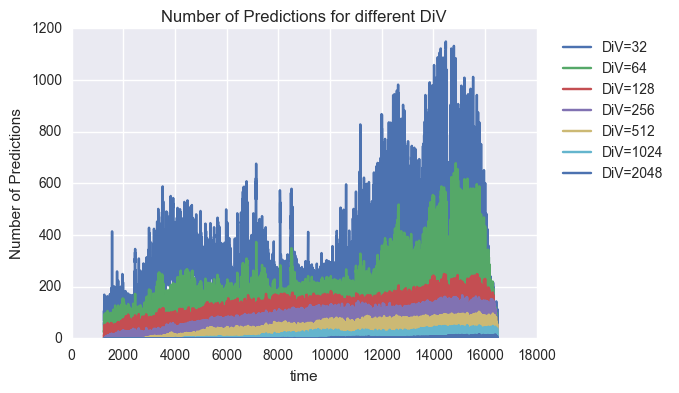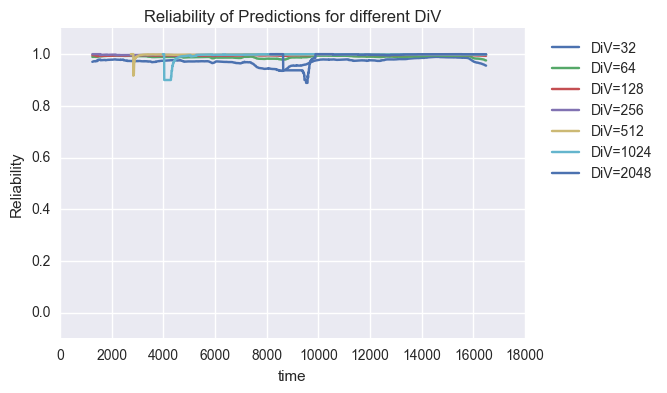(Note that the decreasing number of predictions at the end of the sample is due to the fact that we only count predictions that could be evaluated within the sample. So at the end of the sample the number of preditictions that needs to be evaluated outside the sample increases.)

We see that the number of predictions at every point in time with laws of high quality ($DiV>256$) starts to rise after an initial learning period. It takes time for the laws to collect verifications. By looking at the number of predictions made at a single point in time we can approximately infer the number of laws of a certain quality found at this point in time. For example at the end of the learning period we have found 8 laws that were more than 2048 times verified.

Further we see that predictions with these laws are seldomly false. Most of the predicted relations were $T$ periods later observed. We can give substance to this finding by searching for "meta laws":

In :
calc_meta_laws_1("bike",0.95,["64"])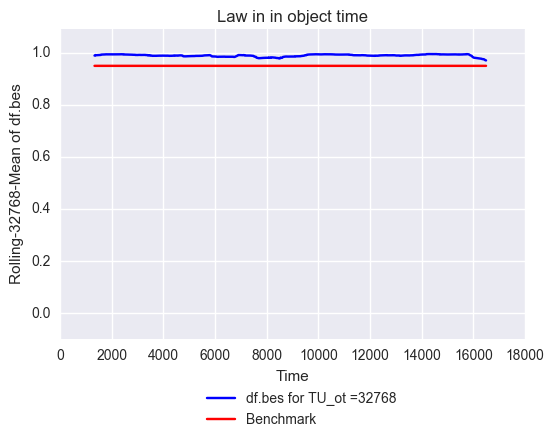Out:
Relation Div_Laws Div_MetaLaws TU_ot
0 Law >= 0.95 64 82.235107 32768.0

It is easy to see that the meta law:

"In each sequence of $TU_{ot}=32768$ predictions the Reliabilty for predictions made with laws of DiV=64 was always greater than 0.95"

was true for each point in time. This meta law was itsself $DiV_{MetaLaw}=82.2$ times verified.

We can calculate $TU_{ot}$ and $DiV_{MetaLaw}$ for multiple DiV-classes and get the following results:

In :
calc_meta_laws_1("bike",0.95)

Out:
Relation Div_Laws Div_MetaLaws TU_ot
0 Law <= 0.95 1 8874.387505 5378.0
1 Law <= 0.95 2 5336.341064 4096.0
2 Law <= 0.95 4 4878.577637 4096.0
3 Law <= 0.95 8 1791.665161 8192.0
4 Law <= 0.95 16 3.193418 2097152.0
5 Law >= 0.95 32 8.300034 524288.0
6 Law >= 0.95 64 82.235107 32768.0
7 Law >= 0.95 128 5892.257028 249.0
8 Law >= 0.95 256 4572.946108 167.0
9 Law >= 0.95 512 2978.582524 103.0
10 Law >= 0.95 1024 1736.274510 51.0
11 Law >= 0.95 2048 450.343750 32.0

We see that up to a DiV of 16 the Rel was always emergent smaller than 0.95 and for $DiV>=32$ there always existed a $TU_{ot}$ for which the reliability of $DiV>=32$-group of laws was for every point in time greater than or equal to 0.95 ($Rel>=0.95$).

We can generalize this finding further by searching over lots of prediction problems and found the general empirical meta law:

"For DiV>256 there always existed a $TU_{ot}$ for which the Reliability of the law group was always $Rel>=0.95$"

### 1.2 Prediction tasks with stable meta laws about temporary regularities (fashions)¶

But we also found prediction problems without laws with $DiV>256$.

We think these precition tasks are systematically different and are identifiable by the above rule.

For example if we try to predict the winnings from betting on the foreign team in games of the major european soccer leagues:

soccer
In this dataset the results of games in the major european soccer leagues and some additional information is given. It can be downloaded from this website: https://www.kaggle.com/hugomathien/soccer.

In :
#plothyp(example,window for rolling_mean,DiV_greater_than,min_Div)
plothyp("soccer_wgaus",1250,False,256)
plotrel("soccer_wgaus",1250,False,256)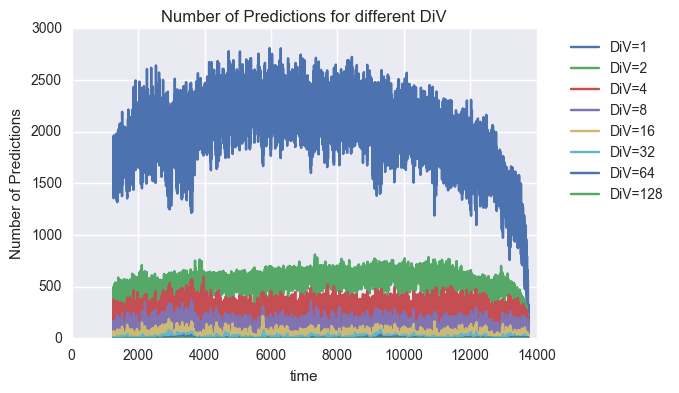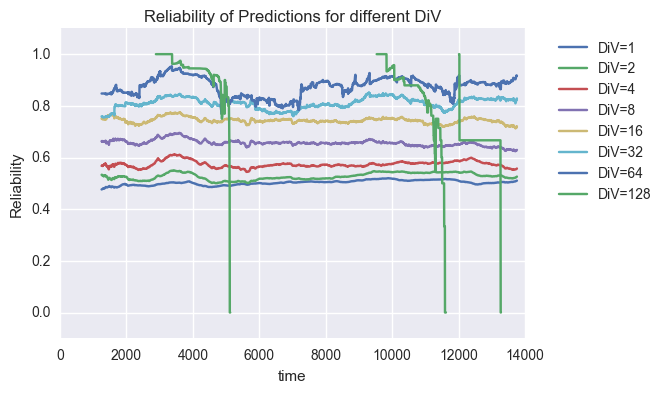(Note that the decreasing number of predictions at the end of the sample is due to the fact that we only count predictions that could be evaluated within the sample. So at the end of the sample the number of preditictions that needs to be evaluated outside the sample encreases.)

We notice first that we can not find laws with a $DiV$ higher than 128. We see that laws tend to be falsified when they reach a DiV of 128. So the laws are temporary in nature. They only exist for a short period in time. But we can see that the found "temporary laws" can help to forecast the relation of the bet winnings of different betting strategies defined by the compared selection rules.
The selection rules in this prediction task can also be interpreted as betting (decision) strategies and we can see whether it was a good idea to predict the relative performance of betting strategies by their preceding relative success. So it seems to be a good idea to follow the strategies that performed best in the immediate past.

Again we can give substance to the above statement by searching for meta laws.

We find laws about the relative reliability by searching for the number of predictions that were necessary until the Rel of predictions with $DiV=2$ was always greater than the Rel for predictions with laws with $DiV=1$ :

In :
calc_meta_laws_2('soccer_wgaus',["1"])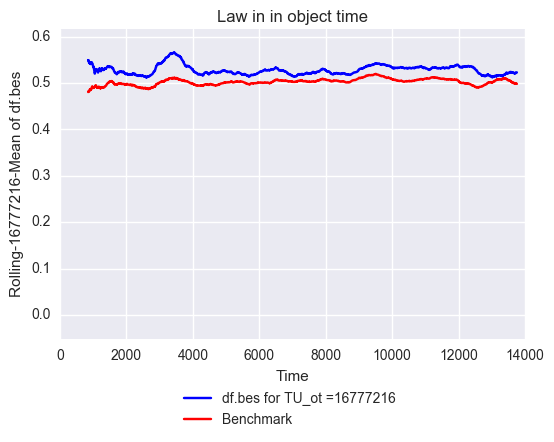Out:
Relation Div_MetaLaws TU_ot
0 Law Rel(2) >= Rel(1) 21.745166 16777216.0

We see that for each sequence of 16777216 bets (note that at each point in time lots of strategies are evaluated) the average winnings of the up to t better temporal strategies would outperform the less verified strategies.

We can evaluate if this relation holds true in general for $Rel(DiV(i))$ and $Rel(DiV(2*i))$

In :
calc_meta_laws_2('soccer_wgaus')

Out:
Relation Div_MetaLaws TU_ot
0 Law Rel(2) >= Rel(1) 21.745166 16777216.0
1 Law Rel(4) >= Rel(2) 22.927415 8388608.0
2 Law Rel(8) >= Rel(4) 67.916649 1048576.0
3 Law Rel(16) >= Rel(8) 79.015491 262144.0
4 Law Rel(32) >= Rel(16) 27.511581 131072.0
5 Law Rel(64) >= Rel(32) 31.770142 8192.0
6 Law Rel(128) >= Rel(64) 0.553223 4096.0

We see that for this prediction problem it was always true that the reliability increases together with the DiV.
In this case it follows as a general advice: "Follow the most verified strategies you can actually find".

But we can also find examples where this advice would be misleading. Let us look at the returns of the Nikkei stock market index. For this example we searched for laws about the relative performance of millions of trading rules. And found the following results:

C_NI
This dataset contains the daily index prices of the Nikkei ranging from 1984 to 2014 for which some additional features (like moving averages) are calculated. For this dataset the percentage return of the next day is the feature of interest and should be predicted.

In :
plothyp("C_NI",1250,False,256)
plotrel("C_NI",1250,False,256)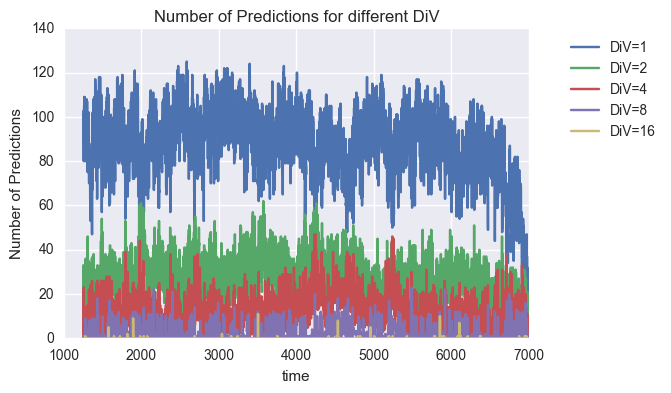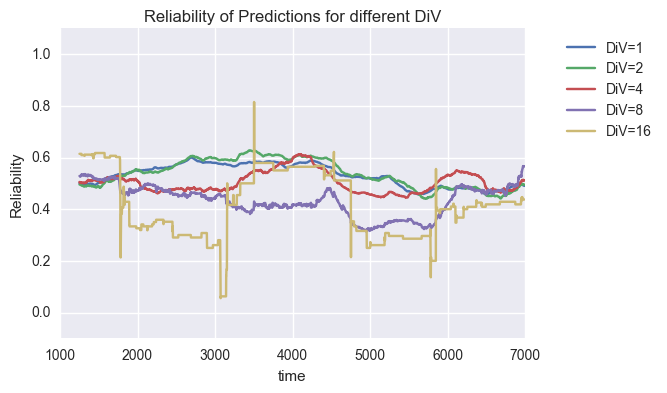The most interesting result is that the rate of true predictions of 16 times verified laws about the relative performance of trading strategies is very disappointing. It seems to be the case that it is lower than 50%.

Let us verify if we can find the corresponding meta laws:

In :
calc_meta_laws_1("C_NI",0.5)

Out:
Relation Div_Laws Div_MetaLaws TU_ot
0 Law >= 0.5 1 0.134724 524288.0
1 Law >= 0.5 2 0.374542 131072.0
2 Law not(<=)and not(>=) 0.5 4 NaN NaN
3 Law <= 0.5 8 1.371338 4096.0
4 Law not(<=)and not(>=) 0.5 16 NaN NaN

We see that only newly appearing laws ($DiV<=2$) predict with an emergent reliability of more than 50%. Eight times verified laws pointed always (emergent) in the false direction.

If we evaluate the relative performance of laws from different DiV-classes we get the following result:

In :
calc_meta_laws_2("C_NI")

Out:
Relation Div_MetaLaws TU_ot
0 Law Rel(2) >= Rel(1) 0.374542 131072.0
1 Law Rel(4) <= Rel(2) 1.258179 32768.0
2 Law Rel(8) <= Rel(4) 0.185669 8192.0
3 Law Rel(16) not(<=)and not(>=) Rel(8) NaN NaN

Perhaps these results show that market participants at stock markets are able to identify patterns and trade against these patterns within a shorter time frame.

Looking at the results for S&P 500 index:

C_SU
This dataset contains the daily index prices of the S&P500 ranging from 1885 to 2014 for which some additional features (like moving averages) are calculated. For this dataset the percentage return of the next day is the feature of interest and should be predicted.

In :
plothyp("C_SU",1250,False,256)
plotrel("C_SU",1250,False,256)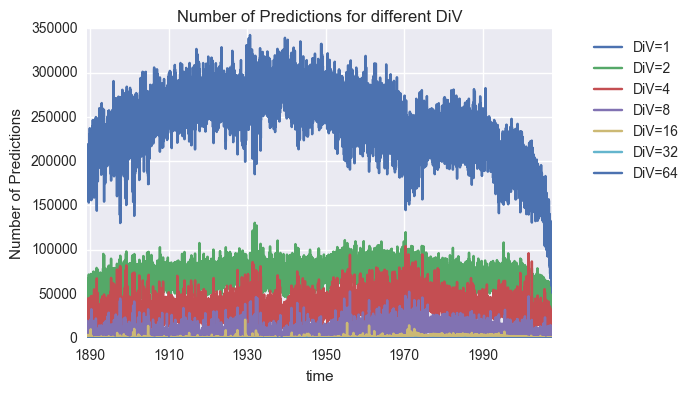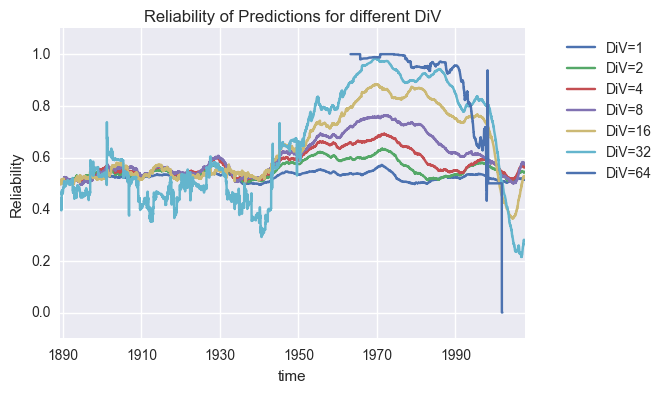We can see that in the period after World War II until the mid 90's the picture is similar to the betting example.

If we take into account that in 1988 Renaissance Technologies started algorithmic trading with the Medallion Fund and in 1997 one of the first electronic communication network for trading stocks (Island ECN) was established the hypothesis seems to be plausible that the change in behaviour of markets is connected to algorithmic capabilities to detect patterns and trade against them.

## 2. Conclusion¶

These results allow an empirical idenfication of three groups of prediction (and decision) problems.

1. Problems where we can rely on a stable set of laws that are seldomly falsified. In standard statistics it is assumed that all problems have this property and we can identify a stable "true model".

2. We can find problems where it is a good idea always to use the laws that "worked" whithin the last period. But the laws that can be found and should be used change over time. The prediction goal is here mainly to identify the pace of change. The time period in which some kind of coordinate behaviour is relatively stable.

3. For some problems it is a good advice not to expect that things continue as in the immediate past. The examples for these problems are mainly from asset markets. There seems to be a possiblity for market participants to use temporary regluarities to exploit other market participants. How this mechanism works exactly is a question of future research.

# Contact¶

If you have any questions or ideas for applications of the presented method, please do not hestitate to contact us via our website.

We are constantly looking for partners interested into applications or further elaborations of the presented method.

## Authors¶

• Hans Frischhut
• Prof. Dr. André Kuck